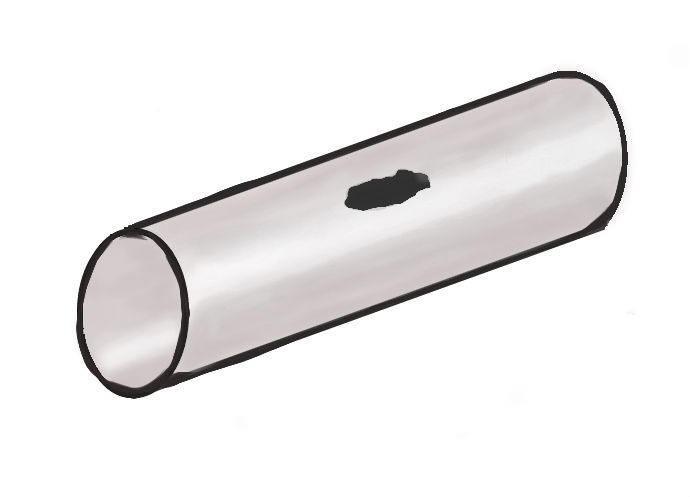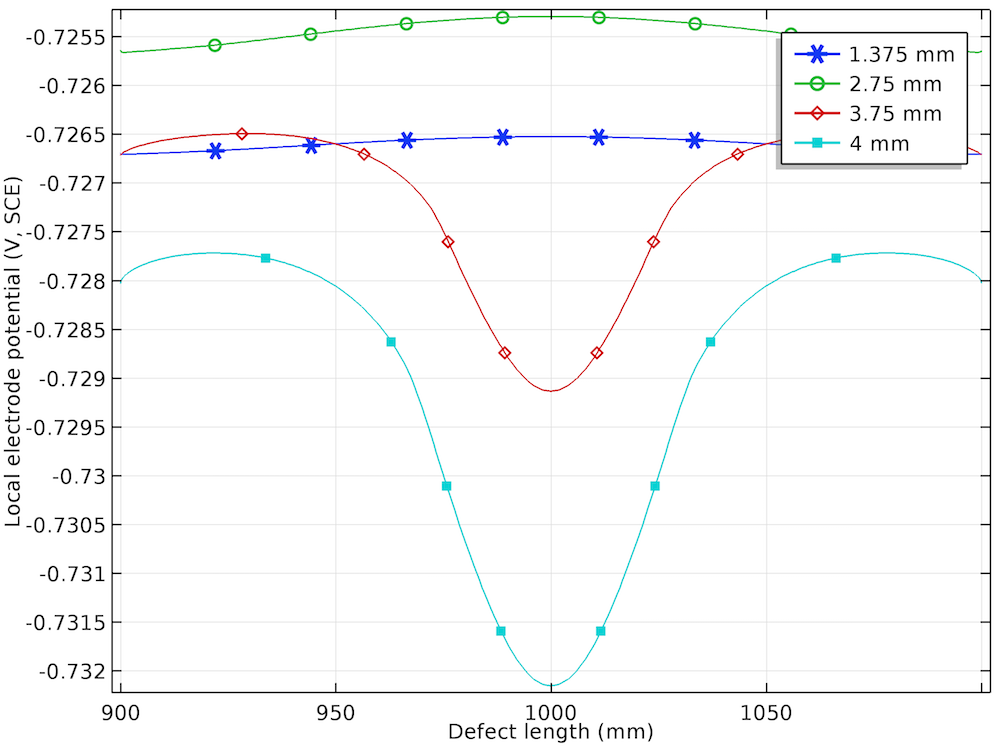# 地下管道应力腐蚀的多物理场模拟

2018年 4月 11日

### 地下管道应力腐蚀### 在 COMSOL Multiphysics® 中模拟应力腐蚀

\sigma_{\textrm{yhard}} = \sigma_{\textrm{exp}} \left( \epsilon_
{\textrm p} + \frac{\sigma_{\textrm{e}}}{E} \right)-\sigma_{\textrm{ys}}

i_{\textrm{a/c}} =\pm i_{\textrm{0,a/c}}10^\frac{\eta_{\textrm{a/c}}}{A_{\textrm{a/c}}}

\eta_{\textrm{a/c}} = \phi_s-\phi_l-E_{\textrm{eq,a/c}}

E_{\textrm{eq,a}} = E_{\textrm{eq0,a}}-\frac{\Delta P_{\textrm m}V_{\textrm m}}{zF}-\frac{TR}{zF}ln \Big(\frac{\nu\alpha}{N_{\textrm 0}}\epsilon_{textrm p}
+1\Big)

i_{\textrm{0,c}} = i_{\textrm{0,c,ref}}10^\frac{-\sigma_{\textrm e}
V_{\textrm m}}{6F A_{c}}

### 仿真结果分析

Von Mises 应力随着指定位移的增大而增大，在腐蚀缺陷的中心达到最大值。在 1.375 mm 和 2.75 mm 的较小指定位移条件下，我们可以看到整个腐蚀缺陷在弹性变形范围内（其中应力低于高强度合金钢的屈服强度 806 Mpa）。在 3.75 mm 和 4 mm 的指定位移条件下，我们可以看到局部应力（特别是腐蚀缺陷中心的局部应力）超过合金钢的屈服强度，这导致缺陷中心发生塑性变形，而缺陷其他区域的变形保持在弹性范围内。### 参考文献

1. L. Y. Xu and Y. F. Cheng, “Development of a finite element model for simulation and prediction of mechanoelectrochemical effect of pipeline corrosion,” Corrosion Science, vol. 73, pp. 150–160, 2013.